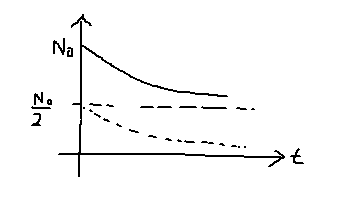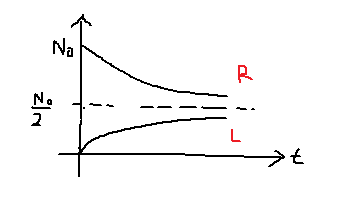# Effusion of particles from one box to another - pressure calculation

## Homework Statement

Suppose we have a container divided into equal halves. Right half is fixed at temperature ##T##, volume ##V_2##.

Initially it has pressure ##P_0##, a hole of area ##A## is opened between them.

Part (a): Show that the pressure in the right half is:

$$p = \frac{p_0}{2} \left[ 1 + exp \left(-\langle v \rangle\frac{A}{V}t \right) \right]$$

Part (b): Vessel has total volume ##10^{-4} m^3##, area of ##7.9 \times 10^{-9} m^2##, ##T = 290 K##. Find the time taken for the pressure on the left half to become half that in the right part.

## The Attempt at a Solution

Part (a)

$$dN = -A\phi_{(t)} dt$$
Using flux as ##\phi = \frac{1}{4} n\langle v \rangle##:
$$dN = - \frac{A}{4} n \langle v \rangle dt = -\frac{A}{4}\left( \frac{N}{V/2} \right) \langle v \rangle dt = -\frac{NA}{2V} \langle v \rangle dt$$

The graph looks like:We solve for the dotted graph, then translate upwards by ##\frac{N_0}{2}##. Integrating, and translating upwards i get: ##p = \frac{p_0}{2} \left[ 1 + exp \left(-\langle v \rangle\frac{A}{2V}t \right) \right]##. Not sure why I'm missing a factor of ##2##..

Part (b)

I know the way to solve this question is to find the pressure on the left half as a function of time, using a process similar to part (a). The graph we want to solve for is (L):But I'm not sure how to begin..

TSny
Homework Helper
Gold Member
The hole is not a one-way passage. Particles can pass through the hole from either side.

The hole is not a one-way passage. Particles can pass through the hole from either side.

Yes but I think that makes the problem insanely difficult. I think we can assume molecules only go from right to left.

Part (a): Any idea why I'm missing by a factor of 2?

Part (b): I don't even know how to start..

TSny
Homework Helper
Gold Member
Surely particles will need to come back through the hole from left to right. Otherwise, the entire gas would eventually end up in the left half.

I get the correct factor of 2 if I account for particles passing back through the hole from left to right. However, I did assume that the gas in the left side is always in thermal equilibrium with the gas in the right side. That would be the case if the wall separating the two halves is a good diathermal (heat conducting) wall.

I think part (b) is just a fairly direct application of the result from part (a). But I haven't worked out the numerical answer.

[EDIT: It seems to me you need to know the molecular mass to answer (b).]

Last edited:
Surely particles will need to come back through the hole from left to right. Otherwise, the entire gas would eventually end up in the left half.

I get the correct factor of 2 if I account for particles passing back through the hole from left to right. However, I did assume that the gas in the left side is always in thermal equilibrium with the gas in the right side. That would be the case if the wall separating the two halves is a good diathermal (heat conducting) wall.

I think part (b) is just a fairly direct application of the result from part (a). But I haven't worked out the numerical answer.

[EDIT: It seems to me you need to know the molecular mass to answer (b).]

Part (a)

$$dN_r = -A\phi_r dt + A \phi_l dt$$
$$= -\frac{AN_r \langle v \rangle}{2V} dt + \frac{AN_l \langle v_l \rangle }{2V} dt$$
$$= -\frac{AN_r \langle v \rangle}{2V} dt + \frac{A(N_0 - N_r) \langle v_l \rangle }{2V} dt$$

Assuming ##\langle v \rangle_l = \langle v \rangle_r## here (If not, ##T_r = \frac{4}{3} T_l##, so ##\langle v \rangle_l = \frac{2}{\sqrt 3} \langle v \rangle_r##):

$$dN = -\frac{A \langle v \rangle}{V} \left(\frac{N_0}{2} - N \right) dt$$

Integrating, I get ## N = \frac{N_0}{2} \left( 1 + e^{-\frac{A \langle v \rangle t}{V}} \right)##. Assuming temperature in the right side stays constant throughout,

$$p = \frac{p_0}{2} \left[ 1 + exp \left(-\langle v \rangle\frac{A}{V}t \right) \right]$$

Part(b)

I can say ##N_{left} = N_0 - N_{right} = \frac{N_0}{2} \left( 1 - e^{-\frac{A\langle v \rangle}{V} t} \right)##.

Thus,

$$\frac{P_{left}}{T_{left}} = \frac{P_0}{2T_0}\left( 1 - e^{-\frac{A\langle v \rangle}{V} t} \right)$$

Now, ##T_{left} = T_{right}## (Assumption made in part (a))

$$P_{left} = \frac{P_0}{2} \left( 1 - e^{-\frac{A\langle v \rangle}{V} t} \right)$$

Mass of nitrogen molecule is ## 4.65 \times 10^{-26} kg##
Average speed is ##468 ms^{-1}##
I get an answer of ##29.7 s##

Last edited:
TSny
Homework Helper
Gold Member
That all looks good.

•1 person